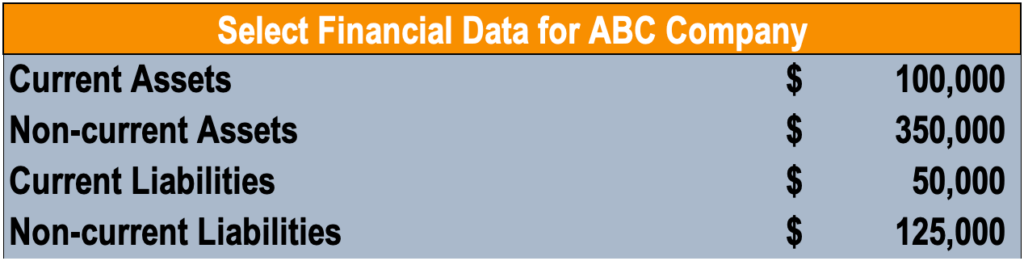# Capital Employed

The capital used by a company to generate profits

## What is Capital Employed?

Capital employed refers to the amount of capital investment needed for a business to operate and provides an indication of how a company is investing its money. Although capital employed can be defined in different contexts, it generally refers to the capital utilized by the company to generate profits. The figure is commonly used in the Return on Capital Employed (ROCE) ratio to measure a company’s profitability and efficiency of capital.### Formula for Capital Employed

Capital employed can be calculated in two ways:

##### Capital Employed = Total Assets – Current Liabilities

Where:

• Total Assets are the total book value of all assets.
• Current Liabilities are liabilities due within a year.

or,

##### Capital Employed = Fixed Assets + Working Capital

Where:

• Fixed Assets, also known as capital assets, are assets that are purchased for long-term use and are vital to the operations of the company. Examples are property, plant, and equipment (PP&E).
• Working Capital is the capital available for daily operations and is calculated as current assets minus current liabilities.

Note: The formula chosen above should be consistently applied (do not switch between formulas when conducting trend analysis or peer comparisons) as the capital employed calculation differs depending on which formula is used. Generally, total assets minus current liabilities is the most commonly used formula in calculating capital employed.

### Sample Calculation

Mary is looking to calculate the capital employed of ABC Company, compiling the following information:Using the first formula above, Mary calculates the total amount as follows:

### Interpreting Capital Employed

Capital employed provides an insight into how a company is investing its money to generate profits. Although the figure varies depending on the formula used, the underlying idea remains the same.

Despite the fact that capital employed provides insight into the capital investment needed for a business to operate, the number in itself is seldom used by analysts. It is commonly used in conjunction with earnings before interest and tax (EBIT) in the return on capital employed (ROCE) ratio. As will be explained below, ROCE is a commonly used ratio by analysts for assessing the profitability of a company for the amount of capital used.

### Return on Capital Employed

Return on capital employed (ROCE) is a profitability ratio that measures the profitability and efficiency to which a company is using its capital. The ROCE is considered one of the best profitability ratios as it shows the operating income generated per dollar invested in capital. The formula for return on capital employed is as follows:

### Example of ROCE

Recall that the capital employed for ABC Company in our example above is \$400,000. Assuming that earnings before interest and taxes of ABC Company is \$30,000, what is the ROCE?

##### ROCE = \$30,000 / \$400,000 = 0.075 = 7.5%

For every dollar invested in its capital, ABC Company generated 7.5 cents in operating income.

CFI offers the Financial Modeling & Valuation Analyst (FMVA)™ certification program for those looking to take their careers to the next level. To keep learning and advancing your career, the following resources will be helpful:

• EBIT vs EBITDA
• Return on Total Capital
• Types of Assets
• Types of Liabilities

### Financial Analyst Training

Get world-class financial training with CFI’s online certified financial analyst training program!

Gain the confidence you need to move up the ladder in a high powered corporate finance career path.

Learn financial modeling and valuation in Excel the easy way, with step-by-step training.